# Evaluating rotation diffusion properties of molecules from short trajectories†

Antonino Polimeno* and Mirco Zerbetto
Università degli Studi di Padova – Dipartimento di Scienze Chimiche, Padova, Italy. E-mail: antonino.polimeno@unipd.it

Received 31st July 2018 , Accepted 6th November 2018

First published on 6th November 2018

We show that under proper assumptions it is possible to estimate with good precision the principal values of the rotational diffusion tensor of proteins from the analysis of short (up to 2–3 ns) molecular dynamics trajectories. We apply this analysis to a few model cases: three polyalanine peptides (2, 5, and 10 aminoacids), the fragment B3 of protein G (GB3), the bovine pancreatic trypsin inhibitor (BPTI), the hen egg-white lysozyme (LYS), the B1 domain of plexin (PB1), and thrombin. The protocol is based on the analysis of the global angular momentum autocorrelation functions, complementing the standard approach based on rotational autocorrelation functions, which requires much longer trajectories. A comparison with values predicted by hydrodynamic modeling and available experimental data is presented.

## 1 Introduction

Transport properties of molecules, and in particular of proteins, are relevant to model and interpret their chemical–physical activity in solution. In the past a number of experimental and theoretical methodologies have been used in order to measure this important parameter. Relevant experimental spectroscopic techniques for instance are fluorescence polarization,1 dichroism,2 dynamic light scattering3 and nuclear magnetic resonance.4 Notice that the measure of tensors describing rotation dissipative properties, like a friction or diffusion tensor, requires a careful definition of reference frames to which the motions can be referred. These tensors are in turn related to specific molecular properties, with respect to which spectroscopic observables are measured. In general, some geometrical considerations are needed in order to set-up a dynamic model, with a set of observables amenable to experimental measurement. To this aim, one usually defines at least the following frames of reference, as shown in Fig. 2: (i) a laboratory frame (LF), i.e. a fixed external frame; (ii) a molecular frame (MF), i.e. a frame fixed on the molecule, where the exact way of defining the MF is actually model-dependent; (iii) an interaction frame (μF), i.e. a local frame linked to the MF where some specific second rank tensor spectroscopic property μ is well-represented. This could be for instance frame where the 15N–1H dipolar tensor is diagonal or a 15N chemical shift (CSA) tensor is diagonal (Fig. 1).5,6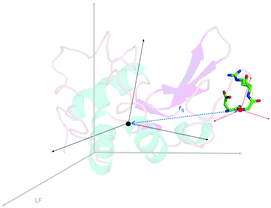Fig. 1 Scheme of reference frames, structure and an example of local frames for GB3.

Computationally, the determination of rotation dissipative properties can be based essentially on two approaches, which complement each other and interpret experimental evidences. On one side, several hydrodynamics approaches have been implemented,7–10 which are capable of good predictive performances at the cost of few free parameters (usually an effective radius of spheres representing atoms or groups of atoms). On the other side, a well known method is based on the extraction of diffusion/friction properties from molecular dynamics (MD) trajectories. Usually, the standard methodology employed to this purpose is based on the analysis of rotation autocorrelation functions.11,12 Both methods are based on the assumptions that the rotational motion is diffusive, i.e. that the molecule can be described as a Brownian diffusive rotator: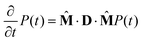(1)
here P(t) ≡ P(Ω,t) is the conditional probability for orientation (Euler angles)Ω at time t,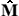is generator of infinitesimal rotations, proportional to the rigid body angular momentum operator and D is the rotational diffusion tensor. In the principal frame of reference (which is usually taken as the MF), which diagonalizes the rotational diffusion tensor, the autocorrelation functions of Wigner matrices13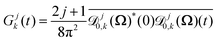(2)
can be used to access the principal components of the diffusion tensor. In the practice, when analyzing MD trajectories, one usually selects a vector, which is pointing to direction n(t), on the protein. The vector is arbitrary and is usually built over the most rigid residues of the protein to better resemble the motion of a rigid rotator. Then, the autocorrelation functions to be calculated are12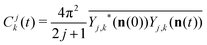(3)
where Yj,k(n(t)) =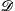j0,k((Ω)(t)).

In the case of an axial D tensor with principal values in the MF D, D, D, eqn (1) and (2) allow to write

 Gjk(t) = e−[D⊥j(j+1)+(D‖−D⊥)k2]t (4)
while, in the common practice of using an arbitrary vector, the MD autocorrelation functions can be interpreted as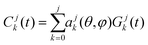(5)
where the coefficients of the expansion (ajk(θ,φ)) are proportional to the real linear combinations of spherical harmonics, calculated on the (θ,φ) polar angles giving the orientation of the chosen vector.12

One well known problem with this direct approach is that in small or medium macromolecule, Gjk(t) has relaxation times of the order of 10 ns, associated with diffusion rates of the order of 106–107 Hz. Therefore one has to calculate quite long molecular dynamics trajectories, of the order of, at least, 100 ns. At present, these long trajectories are affordable, but still the whole process is unnecessarily time consuming. A secondary, but nevertheless not ignorable problem is related to the model of explicit solvent (usually water) used in the simulation. It is known12 that the predicted diffusion tensors strongly depend on the model employed for the water molecules and that the accordance with experimental data scales approximately as the ratio between the MD and real bulk viscosity of water. The worst agreement is found with the more widely employed model of water, i.e. the TIP3P model, which gives, at 298 K, a bulk viscosity about 2.7 times higher than the real viscosity14–16 and the calculated diffusion rates are substantially in the same ratio with experimental measurements. Of course improvements in the force fields employed can significantly abate this issue, which is known to become more relevant with increasing MD trajectory length,11 leading to a wrong sampling of phase space of long-time processes (as diffusive regime properties) and consequently to bad statistics. The purpose of this work is to introduce a slightly alternative approach, which allows to significantly reduce the MD trajectories duration, in order to maximize computational efficiency and accuracy. We propose to calculate the friction (ξ) rotational tensor (see below) instead of the diffusion rotational tensor of a molecule, from observables relaxing with very fast time scales. Components of the conjugate angular momentum are obvious candidates, since they should relax in the sub-picosecond time scale in small/medium size proteins. In order to test the method we analyze eight molecules of increasing size, in particular: 2-alanine (2ALA, 23 atoms), 5-alanine (5ALA, 53 atoms), 10-alanine (10ALA, 103 atoms), the bovine pancreatic trypsin inhibitor (BPTI, 458 atoms), hen egg-white lysozyme (LYS, 1001 atoms), the fragment B3 of protein G (GB3, 437 atoms), the B1 domain of plexin (PB1, 945 atoms), and thrombin (THR, 5031 atoms). The paper is organized as follows: in Section 2 we review the methodology, its rationale, details of the MD simulations and we discuss the results obtained. In Section 3 we summarize our conclusions.

## 2 Method

We assume (i) that the molecule can be considered, at least as a first approximation, a rigid top; (ii) that the rotational relaxation is described by a Fokker–Planck equation,17 including therefore relaxation of the conjugate angular momentum L associated to the reorientation coordinatesΩ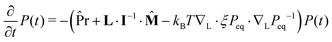(6)
where I is the inertia tensor,ξ is the friction tensor, related to D by the Stokes–Einstein relation D = kT ξ−1, Peq = exp(−H/kBT)/Z is the Boltzmann equilibrium distribution over the energy H = L·I−1·L/2, which is the t → ∞ limit of the conditional probability P(t) ≡ P(Ω,L,t); finally the precessional operator, which is non-zero for generic (non-spherical) top can be written as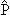r = L × (I−1L × ∇L) (7)

In the following we assume (iii) that precession effects are negligible, which is usually an acceptable approximation in a normal solvent at room temperature (moderate to high friction) and (iv) that tensors I,ξ and hence D are constant diagonal and collinear in the same frame, i.e. the MF. Let us now consider correlation functions of the angular momentum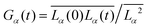(8)
where α = X, Y, Z. As detailed in the ESI, one finds that if precession effects are neglected, Gα(t) is a mono-exponential decay function
 Gα(t) = e−ξαt/Iα = e−t/τα (9)
where the correlation time is τα = 1/ωc,α = Iα/ξα, i.e. the inverse of the collision frequency along the α axis. Therefore, if τα can be obtained, an estimate of the α-component of the diffusion tensor is gained, since Dα = kTτα/Iα, assuming Iα is also known. We can now test this approach by calculating, via MD, correlation functions like (8). For a small to medium weight protein we expect a correlation time to be of the order of 100 fs and therefore the correlation function to relax within 1–10 picoseconds. A 2–3 ns long MD trajectory should be long enough to sample the correlation function.

### 2.1 Simulation details

All the MD trajectories have been calculated in the NPT ensemble, with p = 1 atm, T = 298.15 K for the alanine peptides, T = 300 K for the BPTI, LYS, GB3, and PB1 proteins and T = 310 K for the THR protein. MD trajectories are calculated with the NAMD 2.7b2 package,18 using the CHARMM19 par_all22_prot_cmap force field for all of the molecules but PB1, for which the par_all27_prot_cmap was employed. The oligosaccharide chain of THR was modeled with the chain solution force field (CSFF).20 TIP3P water model was employed in all of the simulations. Counter ions are added in order to neutralize the system. In THR simulations, NaCl was also added up to 0.145 M to reproduce physiological conditions. Temperature coupling was employed to thermostat the system, while a Nosé–Hoover Langevin piston (piston period 200 fs, piston decay 100 fs, piston temperature equal to the thermostat temperature) as the barostat; other parameters are the pair list distance 13.45 Å, non-bonded interactions cutoff 12 Å, with smoothing switch at 10 Å, PME electrostatic. Trajectories were produced from scratch after energy minimization, heating to the target temperature, and system equilibration for RMSD stabilization. Then, 3 ns long production trajectories are calculated, with a time step of integration of 2 fs. Because of the fast time scale of relaxation, molecular conformations must be collected very frequently. In particular, the dumping frequency was every 1 MD time step for the alanine peptides, or every 25 MD time steps for the proteins. The simulation parameters are summarized in Table 1.
Table 1 MD simulation parameters
2ALA 5ALA 10 ALA GB3 BPTI PB1 LYS THR
Protein data bank file 1P7E 6PTI 2JPH 2LZT 1PPB
Total charge 0 0 0 +2 +6 +2 +8 +2
Number of water molecules 898 885 834 3921 2642 10418 6845 21116
Cubic periodic box dimension/Å 30 30 30 50 44 69 60 90
Equilibration period/ns 5 5 5 6 6 6 6 10
Short MD production period/ns 3 3 3 3 3 3 3 3
Long MD production period/ns 200 200 110 100

Results for all of the proteins have been compared with the diffusion tensor based on the decay of rotational correlation functions obtained from long MD trajectories. All simulations, except for LYS, have been obtained with the same setup and software package as described above. The only difference was the sampling frequency, which was 250 MD steps for the small proteins, and 1000 MD steps for thrombin. Literature data have been employed for LYS.

The post-run protocol for the analysis of the MD simulations is the following. First, the snapshots of the dry trajectories are unwrapped (to remove jumps due to the periodic boundary conditions), centered on the center of mass, and aligned along the frame that diagonalizes the tensor of inertia. All atoms, included H atoms, are considered in these operations. At this point, in each of the snapshots, the coordinates of the atoms are referred in the instantaneous inertial molecular frame MF. We stress the importance of using such a frame, since it is the frame where it is assumed thatξ is diagonal. This, in turn, means that only in this frame, in the limit of a rigid rotator, the autocorrelation functions of the components of the angular momentum are expected to be mono exponential decays.

Next, the components of the momenta of the atoms are computed in MF from the numerical forward derivative of the Cartesian coordinates. Finally the three components of the total angular momentum are calculated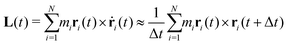(10)
where N is the number of atoms, mi the mass of the i-th atom, Δt the lag time between one snapshot and the next one, and ri(t) the Cartesian coordinates of the i-th atom at time t in the instantaneous frame MF.

In principle, the angular momentum could be calculated from the velocities; however the protocol to express the velocities in MF is more complex than the route described above (e.g., the angular velocity is required). From our tests, the larger efforts needed to use the velocities are not increasing the quality of the results despite the problems that one could expect in performing numerical derivatives. Clearly, it is better to test the stability of the results with the lag time (which controls the quality of the derivatives), but this can be easily done because only one very short trajectory is required for the computation, and the analysis is straightforward.

### 2.2 Analysis

The protocol illustrated in the previous section has been tested on two different sets of molecules. The first set includes the three polyalanine peptides with 2, 5, and 10 aminoacids, and constituted a pilot case-study of the methodology. For the peptides, results are not compared with experimental data; instead, hydrodynamic calculations (see below) are taken as reference. The second set of molecules includes the five proteins GB3, BPTI, PB1, LYS, and THR. Since proteins, as well as macromolecules in general, are the main target of the approach presented here, a more comprehensive comparison is carried out, with results obtained from the standard approach based on rotational autocorrelation functions, and experimental data.

The autocorrelation functions for the three components of the angular momentum showed to be more complex than mono exponential decays. As an example, Fig. 2 shows GX(t), and its logarithmic plot, for the plexin B1 protein (in the ESI, other autocorrelation functions are shown in order to provide a wider picture). They are actually better described by multi-exponential functions. This behavior has been observed in the past also for other observables21 and can be interpreted in terms of coupling of the angular momentum to internal coordinates of the system and/or solvent, that relax at a close timescale. An augmented Fokker–Planck description of a flexible top would be beyond the scope of this work, which is to identify a fast and effective protocol to determine the principal values of D, for an approximately rigid system. Therefore, we adopt the simple strategy of (i) fitting the calculated correlation functions as bi-exponential decays

 Gα(t) = aα2e−t/τα + (1 − aα2)e−t/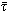α (11)
and (ii) making the assumption that the faster time scale is always associated to the relaxation time of the angular momentum, i.e. to τα. This is compatible with the approximation of considering the protein in the diffusive regime in which the momenta are characterized by correlation times much faster than those of the coordinates. Moreover, in a hydrodynamic interpretation of the friction, the correlation times for the momenta decrease with the size of the portion of the molecule involved in the motion. This makes the global angular momentum (which involves all of the atoms of the protein) a good candidate to show the faster relaxation time scale.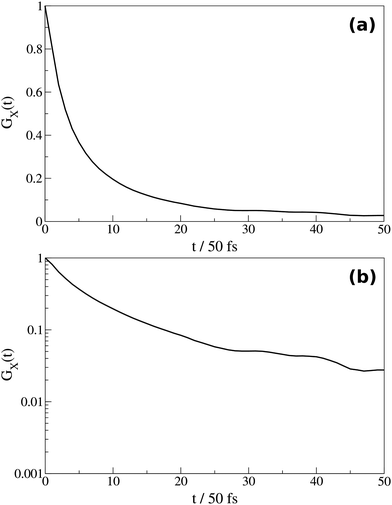Fig. 2 (a) Normalized autocorrelation function, and (b) its logarithmic plot, of LX component of the angular momentum of plexin B1. The logarithmic plot shows that the autocorrelation function is not a simple mono exponential decay.

Results are reported in Table 2. The smaller relaxation time is associated to the relaxation of angular momentum, i.e. τα. In order to calculate friction and diffusion coefficients we consider inertia coefficients averaged over the trajectories. As the table shows, in some cases the autocorrelation functions can be fitted at maximum with one single exponential. This is particular was found for the alanine peptides for which the autocorrelation functions show a damped oscillatory decay due to the closeness in the time scales between the angular momentum and internal motions of the molecule. The correlation functions for the thrombin protein could also be fitted with only one exponential decay. Our interpretation is that in the case of such a large protein, the other relaxation processes are occurring in large time scales, with much less important coupling with the angular momentum. These different behaviours, which are all deviations from the rigid rotator model, reflect different types of couplings of the global angular momentum with other motions, internal to the molecule and/or of the solvent. The origin and rationalization of these effects is not the purpose of this paper. What is interesting is that despite the presence of these effects, the friction/diffusion tensors can be calculated easily and with satisfactory agreement with other methods for the calculation of the diffusion tensor and also with experimental data, as discussed in what follows.

Table 2 Results of fitting of angular momentum autocorrelation functions. Numbers in parenthesis in the averaged inertia coefficients column represent the standard deviation
α a α 2 τ α /fsα /ps I α /10−41 kg m2 ξ α /10−28 kg m2 s−1 D/107 Hz
2ALA
X 1 10.71 0.0016 (0.0002) 0.0052 794
Y 1 12.24 0.0014 (0.0001) 0.0131 315
Z 1 14.66 0.00055 (0.00009) 0.0096 428
5ALA
X 1 14.04 0.012 (0.002) 0.084 48.9
Y 1 13.83 0.010 (0.003) 0.074 55.7
Z 1 10.37 0.0028 (0.0008) 0.027 152
10ALA
X 1 27.83 0.030 (0.001) 0.11 38.1
Y 1 22.38 0.030 (0.002) 0.13 31.2
Z 1 16.94 0.0069 (0.0002) 0.041 102
GB3
X 0.94 152.4 1.34 0.69 (0.03) 0.456 9.1
Y 0.91 155.1 1.09 0.83 (0.03) 0.535 7.7
Z 0.97 177.5 4.95 1.06 (0.05) 0.599 6.9
BPTI
X 0.73 138.2 0.450 0.60 (0.03) 0.432 9.6
Y 0.62 106.9 0.307 1.11 (0.05) 1.03 4.0
Z 0.34 82.3 0.274 1.30 (0.04) 1.58 2.6
PB1
X 0.82 193.0 1.34 2.68 (0.07) 1.39 3.0
Y 0.86 187.5 1.48 3.33 (0.07) 1.78 2.3
Z 0.84 200.7 1.55 3.95 (0.09) 1.97 2.1
LYS
X 0.94 212.3 2.30 2.77 (0.09) 1.30 3.2
Y 0.67 151.1 0.673 3.75 (0.10) 2.48 1.7
Z 0.81 191.8 1.03 4.18 (0.14) 2.18 1.9
THR
X 1 181.0 16.8 (0.2) 9.29 0.46
Y 1 167 15.1 (0.2) 9.06 0.47
Z 1 161 13.1 (0.1) 8.15 0.53

Results obtained from the analysis of the angular momentum correlation functions are here compared with available experimental data, calculations based on rotational autocorrelation functions and a hydrodynamics-based estimate of the rotational diffusion tensor. The latter has been done with the software package DiTe (diffusion tensor):8 in this case calculations are carried out by setting the same temperature of the MD simulations, as reported in the Simulation details subsection; the corresponding viscosity of water is 8.94 mPa s (at 298.15 K), 8.51 mPa s (at 300 K), and 6.92 mPa s (at 310 K). Stick hydrodynamic boundary conditions are imposed. The standard hydrodynamic radius of 2.0 Å has been assigned to all the non-hydrogen atoms of the alinine peptides. In the case of the proteins, the radius has been augmented to 3.4 Å (i.e. by the hydrodynamic radius of water). This increase of the effective hydrodynamic radius is usually necessary for proteins exposing large, high hydrophilic areas to the solvent. Available in the literature is the experimental determination of the isotropic part of the diffusion tensor for the proteins. In particular for GB3, BPTI, PB1, LYS, and THR values of Dexpiso are, respectively, 5.5 × 107 Hz,12 4.3 × 107 Hz,9,11,12 1.9 × 107 Hz,22 2.3 × 107 Hz,9,11,12 and 1.0 × 107 Hz.23

Table 3 shows the comparison between the calculated values from MD with our protocol and with the standard approach based on rotational correlation functions with the experimental values. An overall good agreement with experiments is obtained via the analysis of angular momentum autocorrelation functions, within a factor between 1 and 1.5. The TIP3P model for water molecules has been employed, even if this model reproduces better the bulk properties of water,15,16 rotational diffusion is not always as well reproduced as with the angular momentum based approach. For the case of thrombin a smaller value of the calculated diffusion tensor with respect to the experiment is found. However, we note that both calculations, either based on rotation correlation functions or angular momentum relaxation provide a similar estimate of the diffusion tensor.

The assumption that the inertia and friction/diffusion tensors are approximately collinear has been tested as shown in Fig. 3 where we compare for the 10-alanine peptide and thrombin the orientation of the principal axes of inertia (MF, depicted in red, in the leftmost panels of the figure) with the orientation of the principal values of the diffusion tensor (depicted in green, in the rightmost panels).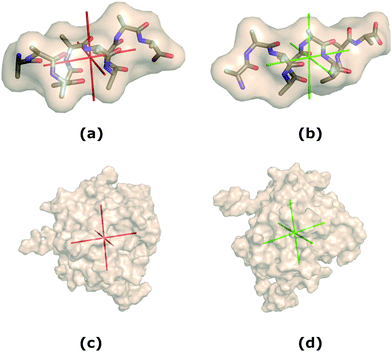Fig. 3 Orientation of (a) the principal axes of inertia of 10-alanine peptide, (b) the principal axes of the diffusion tensor of 10-alanine peptide, (c) the principal axes of inertia of thrombin, and (d) the principal axes of the diffusion tensor of thrombin.

From this analysis we can thus state that the main source of the deviation from the mono exponential decay of the angular momentum autocorrelation functions is the non-rigidity of the protein. However, a fit with a simple mono-exponential or bi-exponential function is able to extract most of the information regarding the relaxation of the total angular momentum, providing a very good estimate of the friction/diffusion tensor from very short MD trajectories.

Finally, the stability of the angular momentum based method, with respect to the length of the trajectory, has been tested. For the GB3, BPTI, and LYS proteins results have been compared with the calculation performed with half of the trajectory. The data is reported in the ESI. It is shown that for the smaller proteins the effect of the trajectory length is more important. A test has been conducted also on the newly included data for thrombin for which the 3 ns short, production MD, was divided in 6 portions of 0.5 ns each. Using only a 0.5 ns portion or the whole trajectory is changing the results <5%, which is comparable with the errors of the fitting procedure, as shown in Table 3. Thus, from our tests, we can say that the precision of our method can be safely set to <10% error.

Table 3 Comparison of available experimental (exp) isotropic part of the rotational diffusion tensor with MD results obtained with analysis of angular momentum (L-MD) and rotational functions available in our laboratory (R-MD) autocorrelation functions, and with the hydrodynamics (DiTe) approach. The polyalanine peptides were compared only with the hydrodynamics based approach. DR-MD for LYS is reported from ref. 12, obtained from a 200 ns production trajectory in the NVE ensemble, with the Amber ff99SB force field for the protein, and SPC/E water model
Molecule D expiso/107 Hz D L-MDiso/107 Hz D R-MDiso/107 Hz D DiTeiso/107 Hz
a In parenthesis we report the error on the diffusion coefficient estimated by propagating the error on the correlation time, which comes from the fitting procedure.
2ALA 516 (2.3%) 442
5ALA 85.5 (2.2%) 113
10ALA 57.1 (3.8%) 69.0
GB3 5.5 7.9 (1.4%) 4.7 5.3
BPTI 4.3 5.4 (7.3%) 3.6 4.7
PB1 1.9 2.5 (1.3%) 1.7 1.9
LYS 2.3 2.3 (2.3%) 3.212 2.5
THR 1.1 0.49 (2.7%) 0.25 1.1

## 3 Conclusions

The proposed method of analysis of short MD trajectories allows to evaluate the principal values of the rotational diffusion tensor of a molecule in a relatively fast and computationally cheap way, via the evaluation of conjugate momentum components autocorrelation functions. Some assumptions are required for the method to be applicable: the first, which is common to all protocols ignoring molecular flexibility, is that the molecule is rigid, or at least can be considered to be in a representative stable conformation for the duration of the simulation, so that geometric fluctuations and large amplitude motions can be neglected. The indication that residual flexibility is present is clearly shown, when evaluating momentum autocorrelation functions, by their multi-exponential decay character, which cannot be reproduced by a simple inertial rigid top model. Nevertheless, we show that by assuming that the fastest decay is associated to rigid rotation, a good estimate of the friction (and diffusion) tensor is still possible. Other minor assumptions are the neglect of precession effects and the assumption of collinearity of the principal frames for the friction, inertia and diffusion tensors. With respect to other MD protocols for estimating the rotational diffusion tensor, based on orientation autocorrelation functions, the main advantage of the method lies in the fact that quite short molecular dynamics simulations are required (2–3 ns vs. 100 ns), still assuring rather accurate estimates of the diffusion tensor components, at the minor cost of a slightly more complex analysis of the trajectories, and an increased storage of data.

A final remark has to be made about the calculation of the angular momentum based on numerical derivatives (eqn (10)). We tested the stability of the results changing the dumping frequency of the short MD trajectories of the proteins from 25 MD steps to 10 MD steps, without noticing differences in the results. This test is suggested in applying the protocol presented, since it takes a short time to be completed, unless the calculation of the angular momentum on MF is performed directly with the velocities obtained in output. In this case, since a roto-translation of the Cartesian coordinates is performed, the transformation of the Cartesian velocities requires that the angular velocity is calculated, as reported in ref. 24.

To conclude, the method presented here provides results of a quality comparable to both the standard analysis of MD trajectories based on rotational correlation functions, and the hydrodynamics-based approach. The latter is extremely fast and reliable, but suffers of the problem of the selection of the effective hydrodynamic radius. While a “standard” value of 2 Å represents a usually good starting point, sometimes the radius needs to be increased to account for interactions with water (e.g., dielectric friction). In these cases, the effective radius is augmented by the hydrodynamic radius of water if the protein is particularly hydrophilic (as was done in this work for the five proteins analysed). This is a ad hoc correction, which limits the predictive capacity of the hydrodynamic method. Besides, a further puzzling problem is the definition of the local viscosity. Usually, but not always, employing the bulk viscosity of the solvent provides good results. In addition, the hydrodynamic boundary conditions, coming from assumptions in the solution of the steady-state Navier–Stokes equations, do not rely directly on the atomistic description of the interaction between solute and solvent. Furthermore, in ordered phases like liquid crystals, the concept of bulk viscosity becomes much more complicated. For all these reasons, it is desirable to employ, when possible, MD simulations. Our method tries to retain the important molecular/atomistic information contained in MD simulations while trying to optimize the computational time of the dissipative properties by choosing conveniently the observable to be computed.

## Conflicts of interest

There are no conflicts to declare.

## Acknowledgements

We thank Prof. Matthias Buck for insightful discussions. Simulations were carried out at the C3P HPC facility of the Department of Chemical Sciences of the University of Padua.

## References

1. G. Belford, R. Belford and G. Weber, Proc. Natl. Acad. Sci. U. S. A., 1972, 69, 1392–1393 CrossRef CAS.
2. W. Wegener, F. Dowben and V. Koester, J. Chem. Phys., 1979, 70, 622–632 CrossRef CAS.
3. K. Schmitz and J. Schurr, Biopolymers, 1973, 12, 1543–1564 CrossRef CAS PubMed.
4. R. Ghose, D. Fushman and D. Cowburn, J. Magn. Reson., 2001, 149, 204–217 CrossRef CAS.
5. A. Abragam, The Principles of Nuclear Magnetism, Clarendon Press, 1961 Search PubMed.
6. J. Peng and G. Wagner, in Investigation of protein motions via relaxation measurements, Academic Press, 1994, pp. 563–595 Search PubMed.
7. G. Moro, Chem. Phys., 1987, 118, 181–197 CrossRef CAS.
8. V. Barone, M. Zerbetto and A. Polimeno, J. Comput. Chem., 2009, 30, 2–13 CrossRef CAS.
9. J. de la Torre, M. Huertas and B. Carrasco, J. Magn. Reson., 2000, 147, 138–146 CrossRef.
10. Y. Ryabov, C. Geraghty, A. Varshney and D. Fushman, J. Am. Chem. Soc., 2006, 128, 15432–15444 CrossRef CAS PubMed.
11. P. E. Smith and W. F. van Gunsteren, J. Mol. Biol., 1994, 236, 629–636 CrossRef CAS.
12. V. Wong and D. Case, J. Phys. Chem. B, 2008, 112, 6013–6024 CrossRef CAS.
13. R. N. Zare, Angular momentum: understanding spatial aspects in chemistry and physics, Wiley, Chichester, 1st edn, 1988 Search PubMed.
14. M. Pekka and N. Lennart, J. Comput. Chem., 2002, 23, 1211–1219 CrossRef.
15. K. Takemura and A. Kitao, J. Phys. Chem. B, 2007, 111, 11870–11872 CrossRef CAS.
16. M. González and J. Abascala, J. Chem. Phys., 2010, 132, 096101 CrossRef.
17. M. Fixman and K. Rider, J. Chem. Phys., 2002, 51, 2425–2438 CrossRef.
18. J. Phillips, R. Braun, W. Wang, J. Gumbart, E. Tajkhorshid, E. Villa, C. Chipot, R. Skeel, L. Kale and K. Schulten, J. Comput. Chem., 2005, 26, 1781–1802 CrossRef CAS.
19. B. Brooks, R. Bruccoleri, B. Olafson, D. States, S. Swaminathan and M. Karplus, J. Comput. Chem., 1983, 4, 187–217 CrossRef CAS.
20. M. Kuttel, J. W. Brady and K. J. Naidoo, J. Comput. Chem., 2002, 23, 1236–1243 CrossRef CAS.
21. T. Mamonova, B. Hespenheide, R. Straub, M. Thorpe and M. Kurnikova, Phys. Biol., 2005, 2, S137–S147 CrossRef CAS PubMed.
22. S. Bouguet-Bonnet and M. Buck, J. Mol. Biol., 2008, 377, 1474–1487 CrossRef CAS.
23. B. Fuglestad, P. M. Gasper, M. Tonelli, J. A. McCammon, P. R. Markwick and E. A. Komives, Biophys. J., 2012, 103, 79–88 CrossRef CAS PubMed.
24. M. B. Hamaneh, L. Zhang and M. Buck, Biophys. J., 2011, 101, 196–204 CrossRef CAS.

### Footnote

Electronic supplementary information (ESI) available. See DOI: 10.1039/c8cp04879g
 This journal is © the Owner Societies 2019